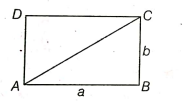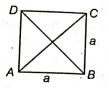## Area

### Introduction

Area:- The area of a plane figure is the total space enclosed by its boundary. It is always expressed in square units.

Perimeter:-  Sum of the length of the border around a plane figure is known as the perimeter of that plane figure.

Rectangle:-  It is a four-sided closed figure with equal opposite sides. Each angle of it is $$90^\circ.$$Area of a Rectangle:-

Area of a rectangleLength x Breadth = ab

Length$$\frac{\textbf { Area }}{\textbf { Breadth }}$$  and

Breadth = $$\frac{\textbf { Area }}{\textbf { Length }}$$

### Perimeter of a Rectangle and Diagonal of a RectanglePerimeter of the rectangle:-

Perimeter of the rectangle  = 2(Length + Breadth)

Diagonal of a Rectangle:- Line segment joining the contrasting corner of the rectangle is known as diagonal of the rectangle. In the adjacent figure, AC is the diagonal of the rectangle.

i.e. Diagonal of a rectangle = $$\sqrt{(\textbf { Length })^{2}+(\textbf { Breadth })^{2}}$$ = $$\sqrt{a^{2}+b^{2}}$$

### Square

Square:-  It is a four-sided closed figure with all sides equal.Area of a Square:-

Area of a square = $$(\textbf { Side } )^{2}$$ = $$(a)^2$$

the side of a square = $$\sqrt{\textbf { Area }}$$

Perimeter of a Square:- Perimeter of a square = 4 x side = 4a

Diagonal of a Square:- Line segment joining the contrasting corner of the square is known as diagonal of the square. In the above figure, AC and BD are the diagonal of square ABCD.

Diagonal of a Square = $$\sqrt{2}$$ x side = $$\sqrt{2}$$a